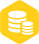# 使用气压传感器MS5611获取温度数据和气压数据

### 描述

MS5611是一款由瑞士公司MEAS推出的高分辨率气压传感器。它具有以下特点：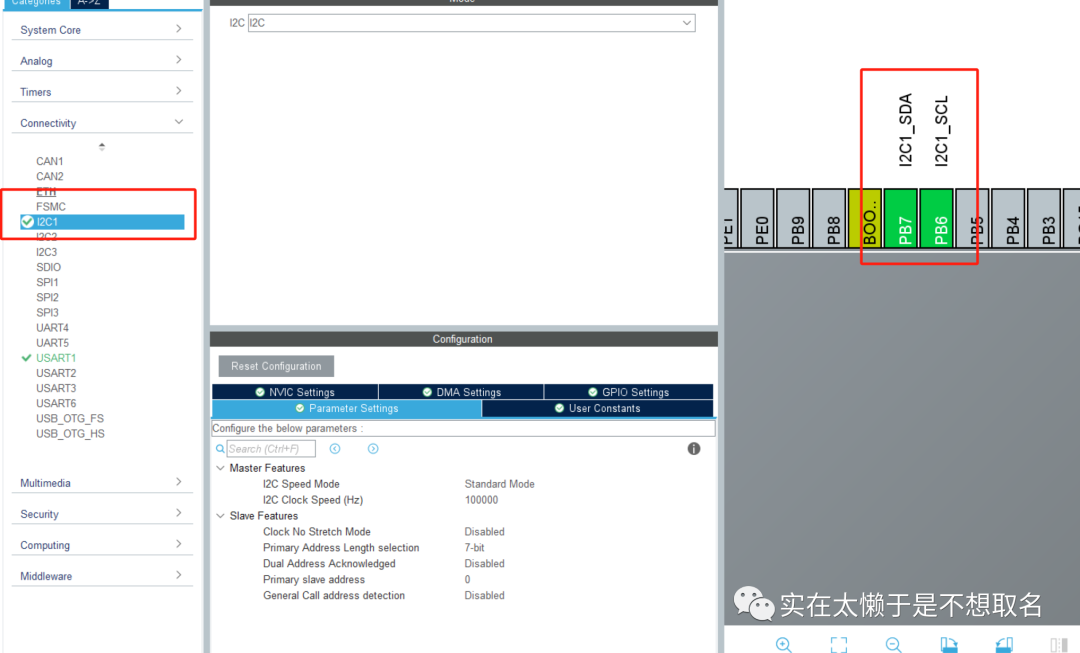``````struct __FILE
{
int handle;
};

struct __FILE __stdout;
//定义_sys_exit()以避免使用半主机模式
void _sys_exit(int x)
{
x = x;
}
//重定义fputc函数
int fputc(int ch, struct __FILE *f)
{
while((USART1- >SR&0X40)==0);//循环发送,直到发送完毕
USART1- >DR = (unsigned char) ch;
return ch;
}
``````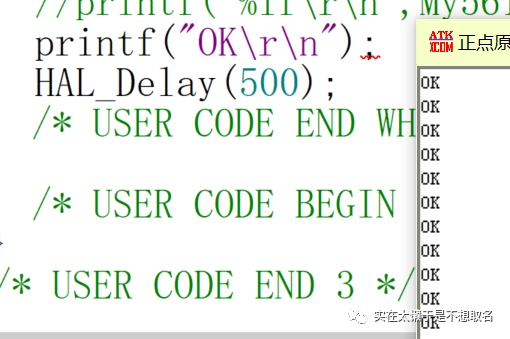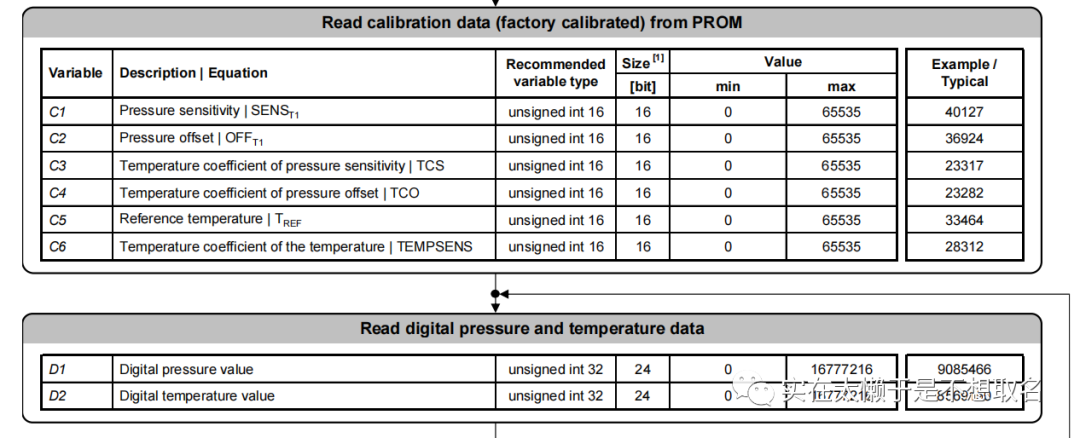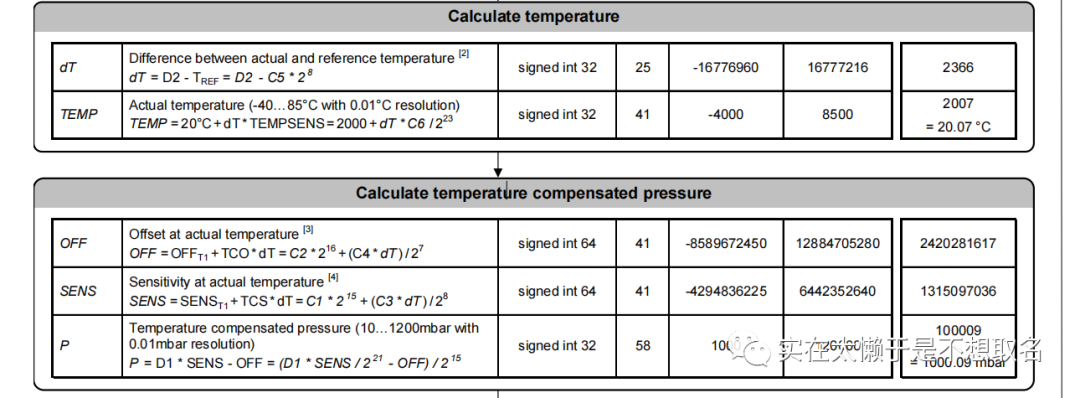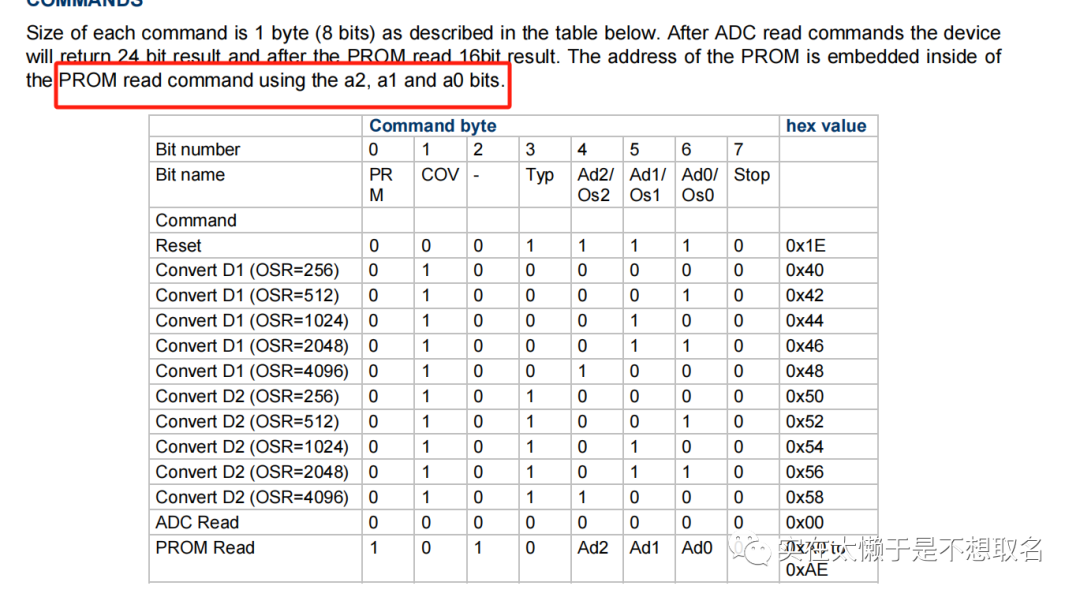``````#define MS5611_I2C_ADDR 0xEE //PS拉高的地址
#define CMD_PROM_RD     0xA0 //PROM基地址

typedef struct {
double temperature;//温度
double pressure;//气压
uint32_t D1;
uint32_t D2;
uint16_t C1;
uint16_t C2;
uint16_t C3;
uint16_t C4;
uint16_t C5;
uint16_t C6;
} MS5611Data;
``````

``````extern I2C_HandleTypeDef hi2c1;
``````

``````HAL_I2C_Mem_Write(&hi2c1, MS5611_I2C_ADDR, CMD_ADC_CONV_D1, I2C_MEMADD_SIZE_8BIT, NULL, 0, 100);
HAL_Delay(10);
uint8_t buffer;
ms5611Data- >D1 = ((uint32_t)buffer < < 16) | ((uint32_t)buffer < < 8) | (uint32_t)buffer;
``````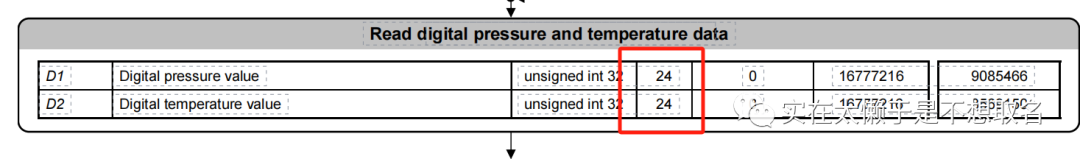``````由于D1,D2都是24个空间，因此我们的数据分3次读取，我们定义一个uint8_t buffer;分别存储三次数据

``````
``````HAL_I2C_Mem_Write(&hi2c1, MS5611_I2C_ADDR, CMD_ADC_CONV_D2, I2C_MEMADD_SIZE_8BIT, NULL, 0, 100);
HAL_Delay(10);
ms5611Data- >D2 = ((uint32_t)buffer < < 16) | ((uint32_t)buffer < < 8) | (uint32_t)buffer;
``````

``````uint8_t coef_data;
for (int i = 1; i <= 6; i++) {
if (i == 1) {
ms5611Data- >C1 = (coef_data < < 8) | coef_data;
} else if (i == 2) {
ms5611Data- >C2 = (coef_data < < 8) | coef_data;
} else if (i == 3) {
ms5611Data- >C3 = (coef_data < < 8) | coef_data;
} else if (i == 4) {
ms5611Data- >C4 = (coef_data < < 8) | coef_data;
} else if (i == 5) {
ms5611Data- >C5 = (coef_data < < 8) | coef_data;
} else if (i == 6) {
ms5611Data- >C6 = (coef_data < < 8) | coef_data;
}
}
``````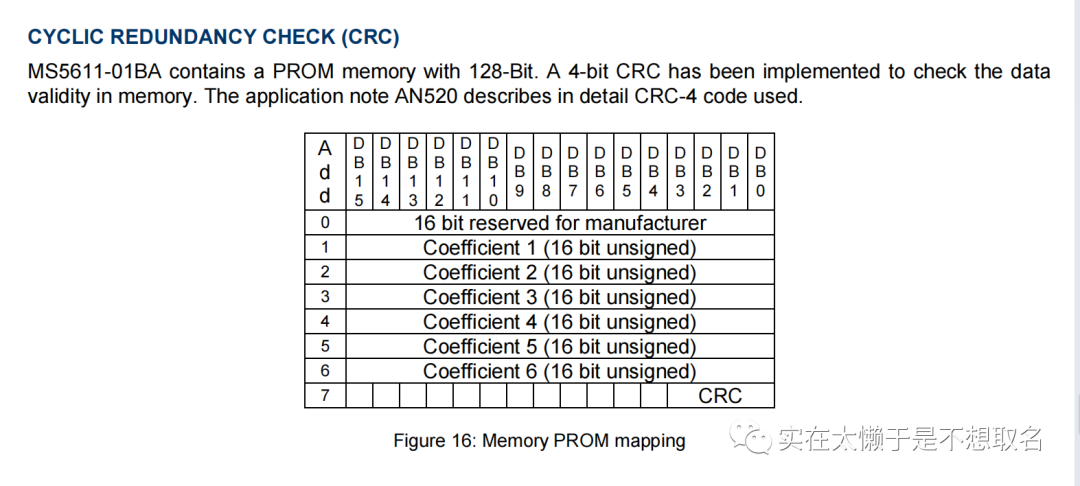``````int32_t dt = ms5611Data- >D2 - ((int32_t)ms5611Data- >C5 *256);
double temp = 2000+((double)dt/ 8388608)*ms5611Data- >C6;
``````

``````int64_t off = ((int64_t)ms5611Data- >C2 * 65536) + ((int64_t)ms5611Data- >C4 * dt) / 128;
int64_t sens = ((int64_t)ms5611Data- >C1 < < 15) + (((int64_t)ms5611Data- >C3 * dt) / (1 < < 8));
double pressure = ((double)ms5611Data- >D1 * sens/2097152 - off) / (1 < < 15);
``````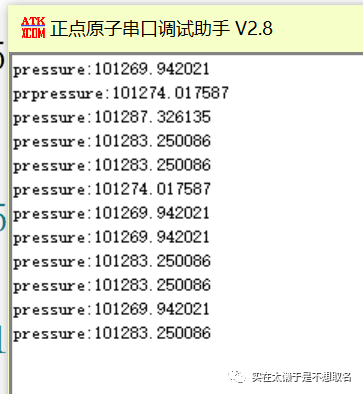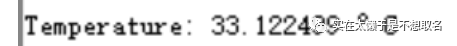×
20